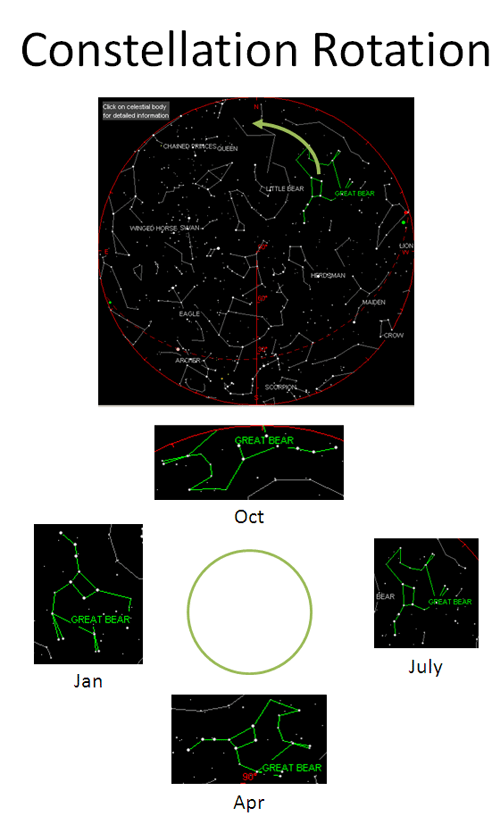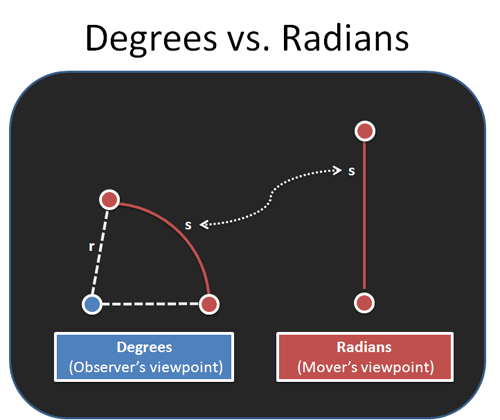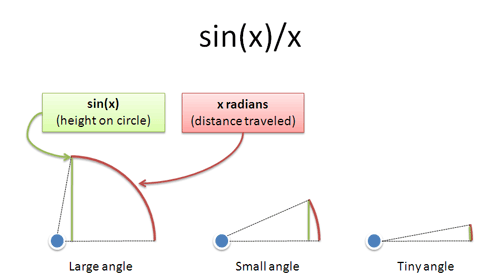﻿ 【数学】角度与弧度的直观理解Angles, Degrees and Radians - 科技一览 - 同义词网 - 同义词网(tongyici.net)

##用户名 Email 自动登录 找回密码 密码 立即注册
 搜索 热搜: 同义词 词根 新概念jason246 发表于 2023-2-2 22:53:14 | 显示全部楼层 |阅读模式It’s an obvious fact that circles should have 360 degrees. Right?

Wrong. Most of us have no idea why there’s 360 degrees in a circle. We memorize a magic number as the “size of a circle” and set ourselves up for confusion when studying advanced math or physics, with their so called “radians”.

“Radians make math easier!” the experts say, without a simple reason why (discussions involving Taylor series are not simple). Today we’ll uncover what radians really are, and the intuitive reason they make math easier.

## Where Do Degrees Come From?

Before numbers and language we had the stars. Ancient civilizations used astronomy to mark the seasons, predict the future, and appease the gods (when making human sacrifices, they’d better be on time).

How is this relevant to angles? Well, bub, riddle me this: isn’t it strange that a circle has 360 degrees and a year has 365 days? And isn’t it weird that constellations just happen to circle the sky during the course of a year?

Unlike a pirate, I bet you landlubbers can’t determine the seasons by the night sky. Here’s the Big Dipper (Great Bear) as seen from New York City in 2008 (try any city):Constellations make a circle every day (video). If you look at the same time every day (midnight), they will also make a circle throughout the year. Here’s a theory about how degrees came to pass:

• Humans noticed that constellations moved in a full circle every year
• Every day, they were off by a tiny bit (“a degree”)
• Since a year has about 360 days, a circle had 360 degrees

But, but… why not 365 degrees in a circle?

Cut ‘em some slack: they had sundials and didn’t know a year should have a convenient 365.242199 degrees like you do.

360 is close enough for government work. It fits nicely into the Babylonian base-60 number system, and divides well (by 2, 3, 4, 6, 10, 12, 15, 30, 45, 90… you get the idea).

## Basing Mathematics on the Sun Seems Perfectly Reasonable

Earth lucked out: ~360 is a great number of days to have in a year. But it does seem arbitrary: on Mars we’d have roughly ~680 degrees in a circle, for the longer Martian year. And in parts of Europe they’ve used gradians, where you divide a circle into 400 pieces.

Many explanations stop here saying, “Well, the degree is arbitrary but we need to pick some number.” Not here: we’ll see that the entire premise of the degree is backwards.

A degree is the amount I, an observer, need to tilt my head to see you, the mover. It’s a tad self-centered, don’t you think?

Suppose you saw a friend go running on a large track:

“Hey Bill, how far did you go?”

“Well, I had a really good pace, I think I went 6 or 7 mile–”

“Shuddup. How far did I turn my head to see you move?”

“What?”

“I’ll use small words for you. Me in middle of track. You ran around. How…much…did…I…turn…my…head?”

“Jerk.”

Selfish, right? That’s how we do math! We write equations in terms of “Hey, how far did I turn my head see that planet/pendulum/wheel move?”. I bet you’ve never bothered to think about the pendulum’s feelings, hopes and dreams.

Do you think the equations of physics should be made simple for the mover or observer?

Much of physics (and life!) involves leaving your reference frame and seeing things from another’s viewpoint. Instead of wondering how far we tilted our heads, consider how far the other person moved.Degrees measure angles by how far we tilted our heads. Radians measure angles by distance traveled.

But absolute distance isn’t that useful, since going 10 miles is a different number of laps depending on the track. So we divide by radius to get a normalized angle:$\displaystyle{\text{Radian} = \frac{\text{distance traveled}}{\text{radius}}}$

You’ll often see this as$\displaystyle{\theta = \frac{s}{r}}$

or angle in radians (theta) is arc length (s) divided by radius (r).

A circle has 360 degrees or 2pi radians — going all the way around is 2 * pi * r / r. So a radian is about 360 /(2 * pi) or 57.3 degrees.

Now don’t be like me, memorizing this thinking “Great, another unit. 57.3 degrees is so weird.” Because it is weird when you’re still thinking about you!

Moving 1 radian (unit) is a perfectly normal distance to travel. Put another way, our idea of a “clean, 90 degree angle” means the mover goes a very unclean pi/2 units. Think about it — “Hey Bill, can you run 90 degrees for me? What’s that? Oh, yeah, that’d be pi/2 miles from your point of view.” The strangeness goes both ways.

Radians are the empathetic way to do math — a shift from away from head tilting and towards the mover’s perspective.

## What’s in a name?

Radians are a count of distance in terms of “radius units”, and I think of “radian” as shorthand for that concept.

Strictly speaking, radians are just a number like 1.5 or 73, and don’t have any units (in the calculation “radians = distance traveled / radius”, we see length is divided by length, so any units would cancel).

But practically speaking, we’re not math robots, and it helps to think of radians as “distance” traveled on a unit circle.

I’m still getting used to thinking in radians. But we encounter the concept of “mover’s distance” quite a bit:

• We use “rotations per minute” not “degrees per second” when measuring certain rotational speeds. This is a shift towards the mover’s reference point (“How many laps has it gone?”) and away from an arbitrary degree measure.

• When a satellite orbits the Earth, we understand its speed in “miles per hour”, not “degrees per hour”. Now divide by the distance to the satellite and you get the orbital speed in radians per hour.

• Sine, that wonderful function, is defined in terms of radians as$\displaystyle{\sin(x) = x - \frac{x^3}{3!} + \frac{x^5}{5!} - \frac{x^7}{7!} ...}$

This formula only works when x is in radians! Why? Well, sine is fundamentally related to distance moved, not head-tilting. But we’ll save that discussion for another day.

## Radian Example 1: Wheels of the Bus

Let’s try a real example: you have a bus with wheels of radius 2 meters (it’s a monster truck bus). I’ll say how fast the wheels are turning and you say how fast the bus is moving. Ready?

“The wheels are turning 2000 degrees per second”. You’d think:

• Ok, the wheels are going 2000 degrees per second. That means it’s turning 2000/360 or 5 and 5/9ths rotations per second. Circumference = 2 * pi * r, so it’s moving, um, 2 * 3.14 * 5 and 5/9ths… where’s my calculator

Ok. Now imagine a car with wheels of radius 2 meters (also a monster). “The car wheels are turning 6 radians per second”. You’d think:

• Radians are distance along a unit circle — we just scale by the real radius to see how far we’ve gone. 6 * 2 = 12 meters per second. Next question.

Wow -- the car was easier to figure out than the bus! No crazy formulas, no pi floating around — just multiply to convert rotational speed to linear speed. All because radians speak in terms of the mover.

The reverse is easy too. Suppose you’re cruising 90 feet per second on the highway (60 miles per hour) on your 24″ inch rims (radius 1 foot). How fast are the wheels turning?

Well, 90 feet per second / 1 foot radius = 90 radians per second.

That was easy. I suspect rappers sing about 24″ rims for this very reason.

Time for a beefier example. Calculus is about many things, and one is what happens when numbers get really big or really small.

When you make x small, like .01, sin(x) gets small as well. And the ratio of sin(x)/x seems to be about .017 — what does that mean? Even stranger, what does it mean to multiply or divide by a degree? Can you have square or cubic degrees?

Radians to the rescue! Knowing they refer to distance traveled (they’re not just a ratio!), we can interpret the equation this way:

• x is how far you traveled along a circle
• sin(x) is how high on the circle you are

So sin(x)/x is the ratio of how high you are to how far you’ve gone: the amount of energy that went in an “upward” direction. If you move vertically, that ratio is 100%. If you move horizontally, that ratio is 0%.When something moves a tiny amount, such as 0 to 1 degree from our perspective, it’s basically going straight up. If you go an even smaller amount, from 0 to .00001 degrees, it’s really going straight up. The distance traveled (x) is very close to the height (sin(x)).

As x shrinks, the ratio gets closer to 100% — more motion is straight up. Radians help us see, intuitively, why sin(x)/x approaches 1 as x gets tiny. We’re just nudging along a tiny amount in a vertical direction. By the way, this also explains why sin(x) ~ x for small numbers.

Sure, you can rigorously prove this using calculus, but the radian intuition helps you understand it.

Remember, these relationships only work when measuring angles with radians. With degrees, you’re comparing your height on a circle (sin(x)) with how far some observer tilted their head (x degrees), and it gets ugly fast.

## So What’s the Point?

Degrees have their place: in our own lives, we’re the focal point and want to see how things affect us. How much do I tilt my telescope, spin my snowboard, or turn my steering wheel?

With natural laws, we’re an observer describing the motion of others. Radians are about them, not us. It took me many years to realize that:

• Degrees are arbitrary because they’re based on the sun (365 days ~ 360 degrees), but they are backwards because they are from the observer’s perspective.
• Because radians are in terms of the mover, equations “click into place”. Converting rotational to linear speed is easy, and ideas like sin(x)/x make sense.

Even angles can be seen from more than one viewpoint, and understanding radians makes math and physics equations more intuitive.

Happy math.

 您需要登录后才可以回帖 登录 | 立即注册 本版积分规则 回帖后跳转到最后一页

GMT+8, 2023-5-29 23:20